2023-05-19

Shengjie Xiu, Yifan Yu, and Daniel P. Palomar
The Hong Kong University of Science and Technology (HKUST)

Welcome to the intradayModel package! This vignette provides an overview of the package’s features and how to use them. intradayModel uses state-space models to model and forecast financial intraday signal, with a focus on intraday trading volume. Our team is currently working on expanding the package to include more support for intraday volatility.

# Quick start

To get started, we load our package and sample data: the 15-minute intraday trading volume of AAPL from 2019-01-02 to 2019-06-28, covering 124 trading days. We use the first 104 trading days for fitting, and the last 20 days for evaluation of forecasting performance.

library(intradayModel)
data(volume_aapl)
volume_aapl[1:5, 1:5] # print the head of data
#>          2019-01-02 2019-01-03 2019-01-04 2019-01-07 2019-01-08
#> 09:30 AM   10142172    3434769   20852127   15463747   14719388
#> 09:45 AM    5691840   19751251   13374784    9962816    9515796
#> 10:00 AM    6240374   14743180   11478596    7453044    6145623
#> 10:15 AM    5273488   14841012   16024512    7270399    6031988
#> 10:30 AM    4587159   18041115    8686059    7130980    5479852

volume_aapl_training <- volume_aapl[, 1:104]
volume_aapl_testing <- volume_aapl[, 105:124]

Next, we fit a univariate state-space model using fit_volume( ) function.

model_fit <- fit_volume(volume_aapl_training)

Once the model is fitted, we can analyze the hidden components of any intraday volume based on all its observations. By calling decompose_volume( ) function with purpose = "analysis", we obtain the smoothed daily, seasonal, and intraday dynamic components. It involves incorporating both past and future observations to refine the state estimates.

analysis_result <- decompose_volume(purpose = "analysis", model_fit, volume_aapl_training)

# visualization
plots <- generate_plots(analysis_result)
plots$log_components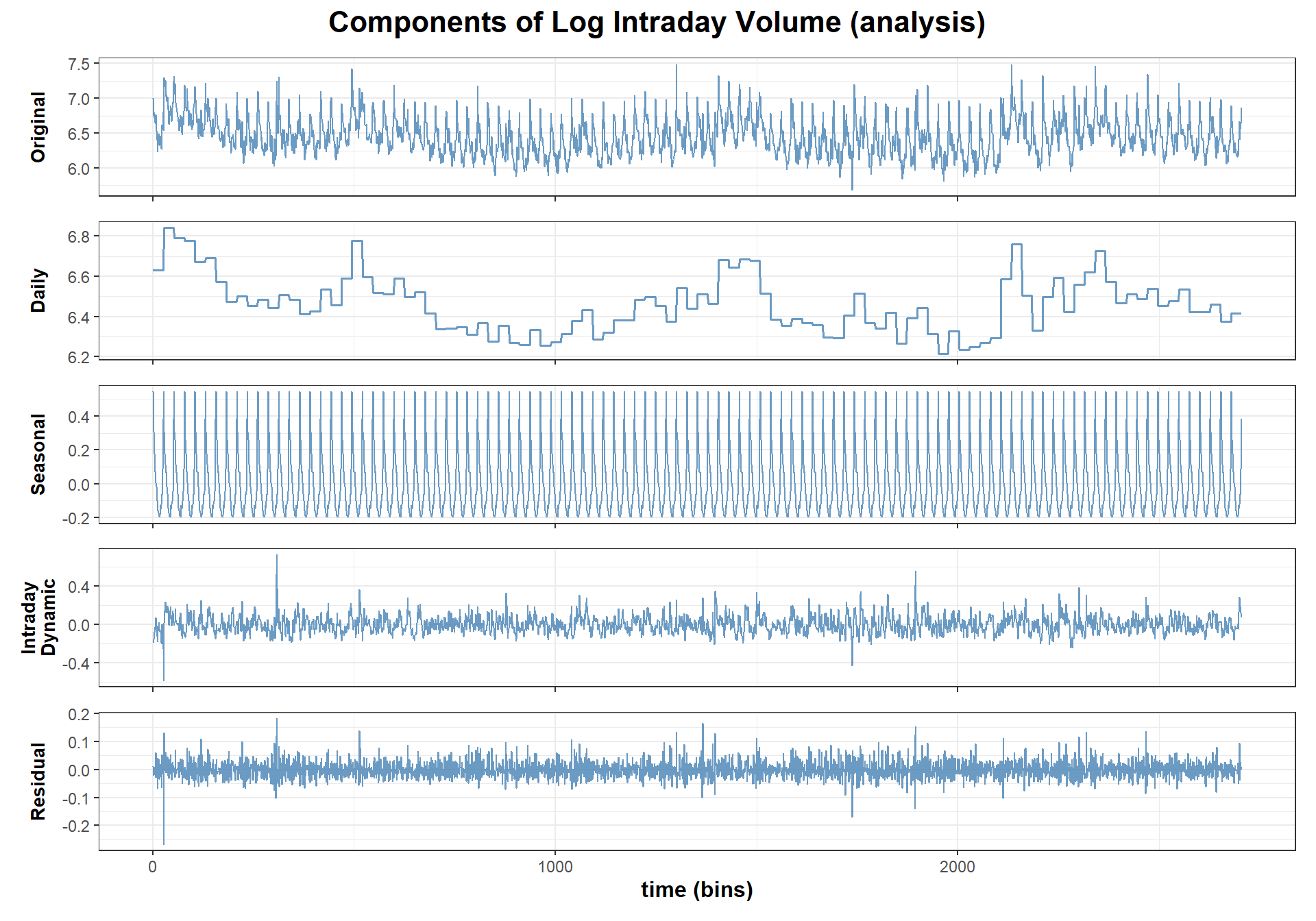To see how well our model performs on new data, we call forecast_volume( ) function to do one-bin-ahead forecast on the testing set. forecast_result <- forecast_volume(model_fit, volume_aapl_testing) # visualization plots <- generate_plots(forecast_result) plots$original_and_forecast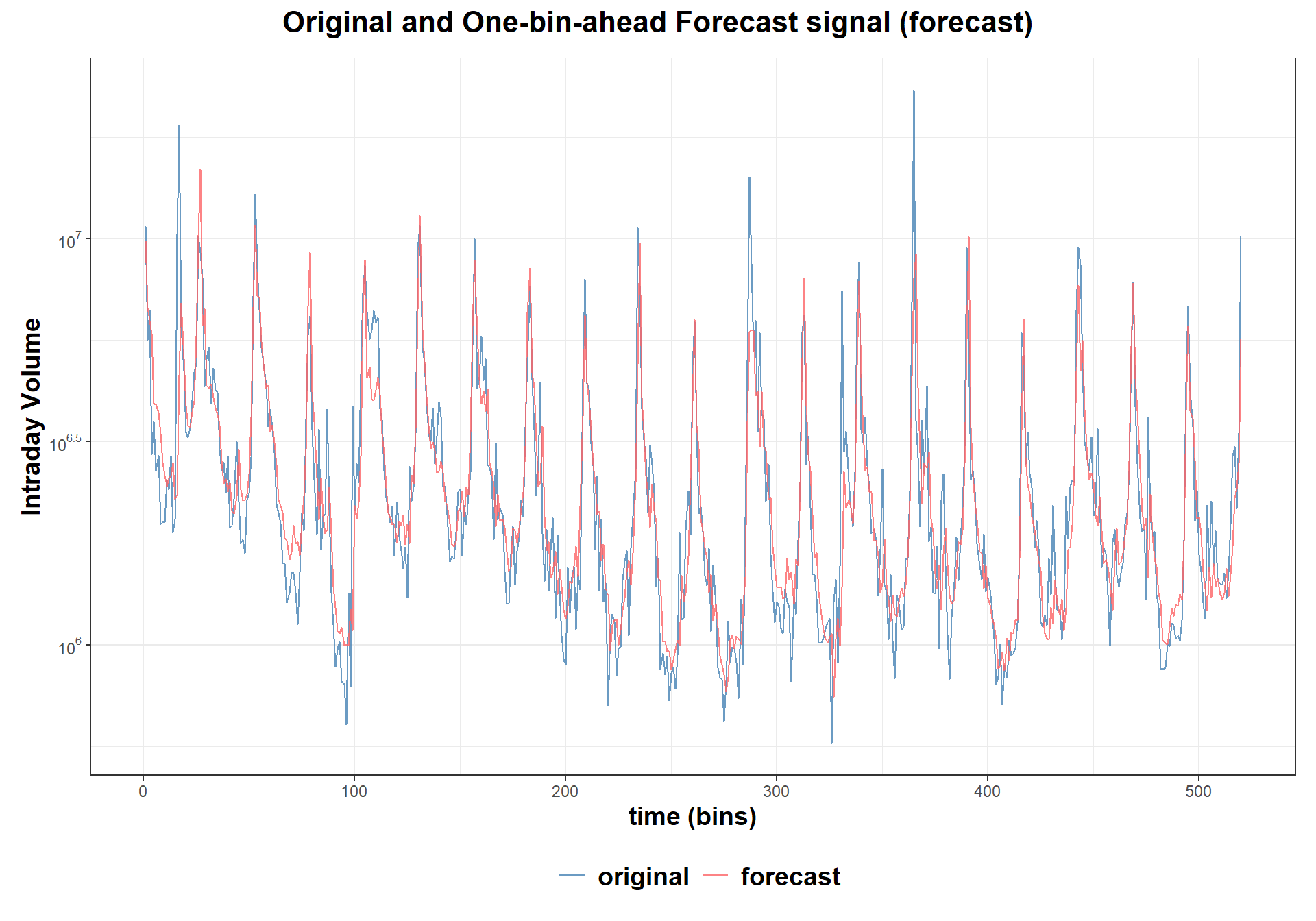Now that you have a quick start on using the package, let’s explore the details and dive deeper into its functionalities and features.

# Usage of the package

## Preliminary theory

Intraday observations of trading volume are divided into days, indexed by $$t\in\{1,\dots,T\}$$. Each day is further divided into bins, indexed by $$i\in\{1,\dots,I\}$$. To refer to a specific observation, we use the index $$\tau = I \times (t-1) + i$$.

Our package uses a state-space model to extract several components of intraday volume. These components include the daily component, which adjusts the mean level of the time series; the seasonal component, which captures the U-shaped intraday periodic pattern; and the intraday dynamic component, which represents movements within a day.

The observed intraday volume can be written in a multiplicative combination of the components :

$\large \text{intraday volume} = \text{daily} \times \text{seasonal} \times \text{intraday dynamic} \times \text{noise}. \tag{1} \small$

Alternatively, by taking the logarithm transform, the intraday volume can be also regarded as an addictive combination of these components:

$\large y_{\tau} = \eta_{\tau} + \phi_i + \mu_{t,i} + v_{t,i}. \tag{2} \small$

The state-space model proposed by is defined on Equation (2) as \large \begin{aligned} \mathbf{x}_{\tau+1} &= \mathbf{A}_{\tau}\mathbf{x}_{\tau} + \mathbf{w}_{\tau},\\ y_{\tau} &= \mathbf{C}\mathbf{x}_{\tau} + \phi_{\tau} + v_\tau, \end{aligned} \tag{3} \small where

• $$\mathbf{x}_{\tau} = [\eta_{\tau}, \mu_{\tau}]^\top$$ is the hidden state vector containing the log daily component and the log intraday dynamic component;

• $$\mathbf{A}_{\tau} = \left[\begin{array}{l}a_{\tau}^{\eta}&0\\0&a^{\mu}\end{array} \right]$$ is the state transition matrix with $$a_{\tau}^{\eta} = \begin{cases}a^{\eta}&\tau = kI, k = 1,2,\dots\\0&\text{otherwise};\end{cases}$$

• $$\mathbf{C} = [1, 1]$$ is the observation matrix;

• $$\phi_{\tau}$$ is the corresponding element from $$\boldsymbol{\phi} = [\phi_1,\dots, \phi_I]^\top$$, which is the log seasonal component;

• $$\mathbf{w}_{\tau} = \left[\epsilon_{\tau}^{\eta},\epsilon_{\tau}^{\mu}\right]^\top \sim \mathcal{N}(\mathbf{0}, \mathbf{Q}_{\tau})$$ represents the i.i.d. Gaussian noise in the state transition, with a time-varying covariance matrix $$\mathbf{Q}_{\tau} = \left[\begin{array}{l}(\sigma_\tau^{\eta})^2&0\\0&(\sigma^{\mu})^2\end{array} \right]$$ and $$\sigma_\tau^{\eta} = \begin{cases}\sigma^{\eta}&\tau = kI, k = 1,2,\dots\\0&\text{otherwise};\end{cases}$$

• $$v_\tau \sim \mathcal{N}(0, r)$$ is the i.i.d. Gaussian noise in the observation;

• $$\mathbf{x}_1$$ is the initial state at $$\tau = 1$$, and it follows $$\mathcal{N}(\mathbf{x}_0, \mathbf{V}_0)$$.

In this model, $$\boldsymbol{\Theta} = \{a^{\eta}, a^{\mu}, (\sigma^{\eta})^2, (\sigma^{\mu})^2, r, \boldsymbol{\phi}, \mathbf{x}_0, \mathbf{V}_0 \}$$ are treated as parameters.

## Datasets

Two data classes of intraday volume are supported:

• a 2D numeric matrix of size (n_bin, n_day);

• an xts object.

To help you get started, we provide two sample datasets: a matrix-class volume_aapl and an xts-class volume_fdx. Here, we elaborate on the later one.

data(volume_fdx)
#>                     FDX.Volume
#> 2019-07-01 09:30:00      78590
#> 2019-07-01 09:45:00      81203
#> 2019-07-01 10:00:00      52789
#> 2019-07-01 10:15:00      54344
#> 2019-07-01 10:30:00      47637
#> 2019-07-01 10:45:00      36240
tail(volume_fdx)
#>                     FDX.Volume
#> 2019-12-31 14:30:00      19284
#> 2019-12-31 14:45:00      18030
#> 2019-12-31 15:00:00      30946
#> 2019-12-31 15:15:00      45762
#> 2019-12-31 15:30:00      72011
#> 2019-12-31 15:45:00     219667

## Fitting

fit_volume(data, fixed_pars = NULL, init_pars = NULL, verbose = 0, control = NULL)

To fit a univariate state-space model on intraday volume, you should use fit_volume( ) function. If you want to fix some parameters to specific values, you can provide a list of values to fixed_pars. If you have prior knowledge of the initial values for the unfitted parameters, you can provide it through init_pars. Besides, verbose controls the level of print, and more control options can be set via control.

The fitting process stops when either the maximum number of iterations is reached or the termination criteria is met $$\|\Delta \boldsymbol{\Theta}_i\| \le \text{abstol}$$.

The following code shows how to fit the model to the FDX stock.

# set fixed value
fixed_pars <- list()
fixed_pars$"x0" <- c(13.33, -0.37) # set initial value init_pars <- list() init_pars$"a_eta" <- 1

volume_fdx_training <- volume_fdx['2019-07-01/2019-11-30']
model_fit <- fit_volume(volume_fdx_training, verbose = 2, control = list(acceleration = TRUE))
#> Warning in intraday_xts_to_matrix(data): For input xts:
#>  Remove trading days with missing bins: 2019-07-03, 2019-11-29.
#> iter:5 diff:0.002073476
#> iter:10 diff:0.003347168
#> iter:15 diff:0.0008842684
#> iter:20 diff:0.001107481
#> iter:25 diff:0.0003287878
#> iter:30 diff:0.0003875934
#> iter:35 diff:0.0001219829
#> Success! abstol test passed at 39 iterations.
#> --- obtained parameters ---
#> List of 8
#>  $a_eta : num 0.999 #>$ a_mu   : num 0.839
#>  $var_eta: num 0.121 #>$ var_mu : num 0.0358
#>  $r : num 0.118 #>$ phi    : num [1:26] 0.8415 0.4275 0.3783 0.216 0.0848 ...
#>  $x0 : num [1:2] 10.899 -0.303 #>$ V0     : num [1:2, 1:2] 6.76e-06 -6.90e-07 -6.90e-07 9.07e-06
#> ---------------------------

Trading days with missing bins are automatically removed. They are 2019-07-03 (Independence Day) and 2019-11-29 (Thanksgiving Day) which have early close.

## Decomposition

decompose_volume(purpose, model, data, burn_in_days = 0)

decompose_volume( ) function allows you to decomposes the intraday volume into its daily, seasonal, and intraday dynamic components.

With purpose = "analysis", it applies Kalman smoothing to estimate the hidden states given all available observations up to a certain point in time. The daily component and intraday dynamic component at time $$\tau$$ are the smoothed state estimate conditioned on all the data, and denoted by $$\mathbb{E}[\mathbf{x}_{\tau}|\{y_{j}\}_{j=1}^{M}]$$, where $$M$$ is the total number of bins in the dataset. Besides, the seasonal component has the value of $$\boldsymbol{\phi}$$.

analysis_result <- decompose_volume(purpose = "analysis", model_fit, volume_fdx_training)
#> Warning in intraday_xts_to_matrix(data): For input xts:
#>  Remove trading days with missing bins: 2019-07-03, 2019-11-29.

str(analysis_result)
#> List of 4
#>  $original_signal : num [1:2730] 78590 81203 52789 54344 47637 ... #>$ smooth_signal    : num [1:2730] 92764 65438 61063 53198 47103 ...
#>  $smooth_components:List of 4 #> ..$ daily   : num [1:2730] 54116 54116 54116 54116 54116 ...
#>   ..$dynamic : num [1:2730] 0.739 0.789 0.773 0.792 0.8 ... #> ..$ seasonal: num [1:2730] 2.32 1.53 1.46 1.24 1.09 ...
#>   ..$residual: num [1:2730] 0.847 1.241 0.865 1.022 1.011 ... #>$ error            :List of 3
#>   ..$mae : num 14233 #> ..$ mape: num 0.223
#>   ..$rmse: num 38111 #> - attr(*, "type")= chr [1:2] "analysis" "smooth" Function generate_plots( ) visualizes the smooth components and the smoothing performance. plots <- generate_plots(analysis_result) plots$log_components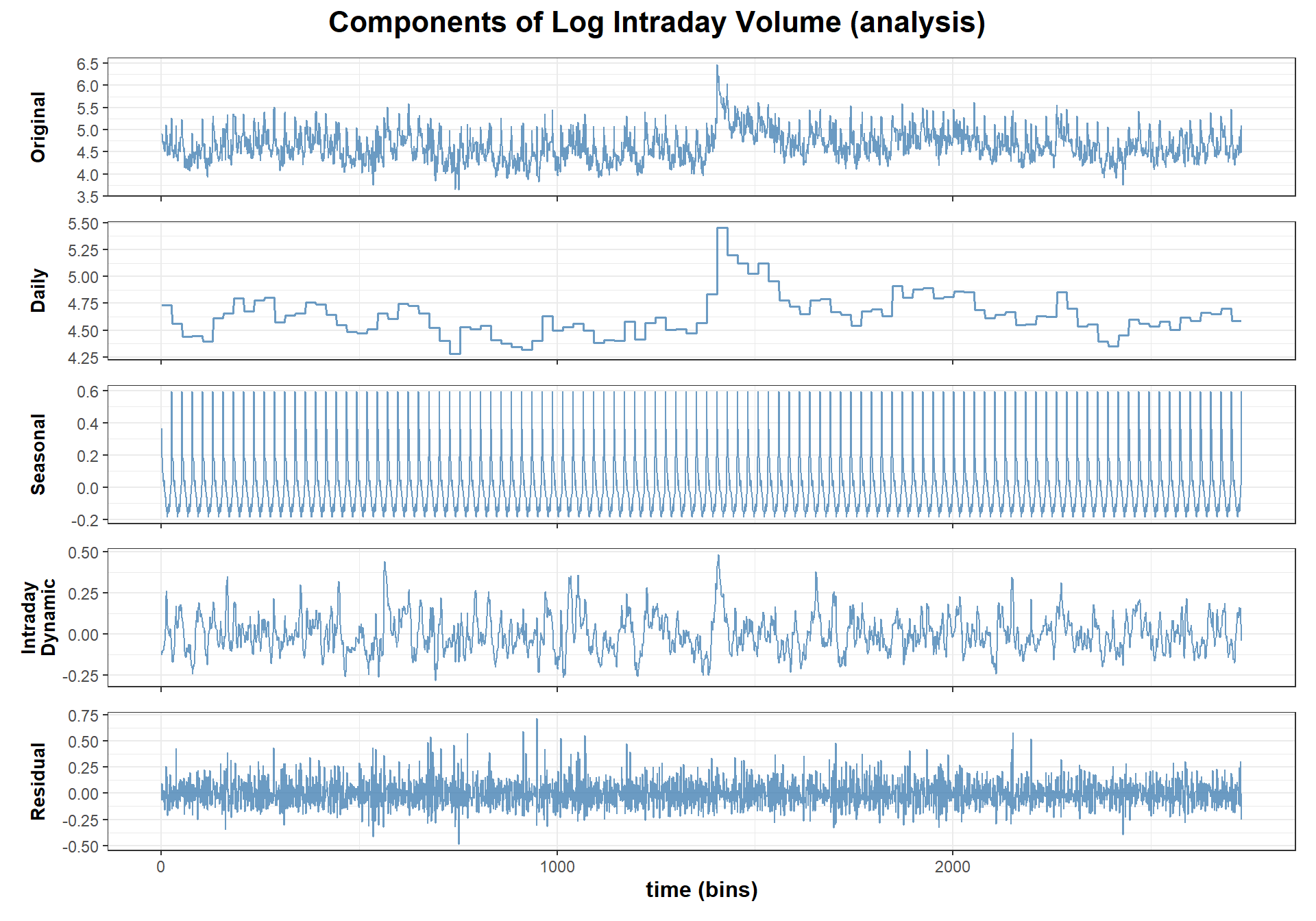plots$original_and_smooth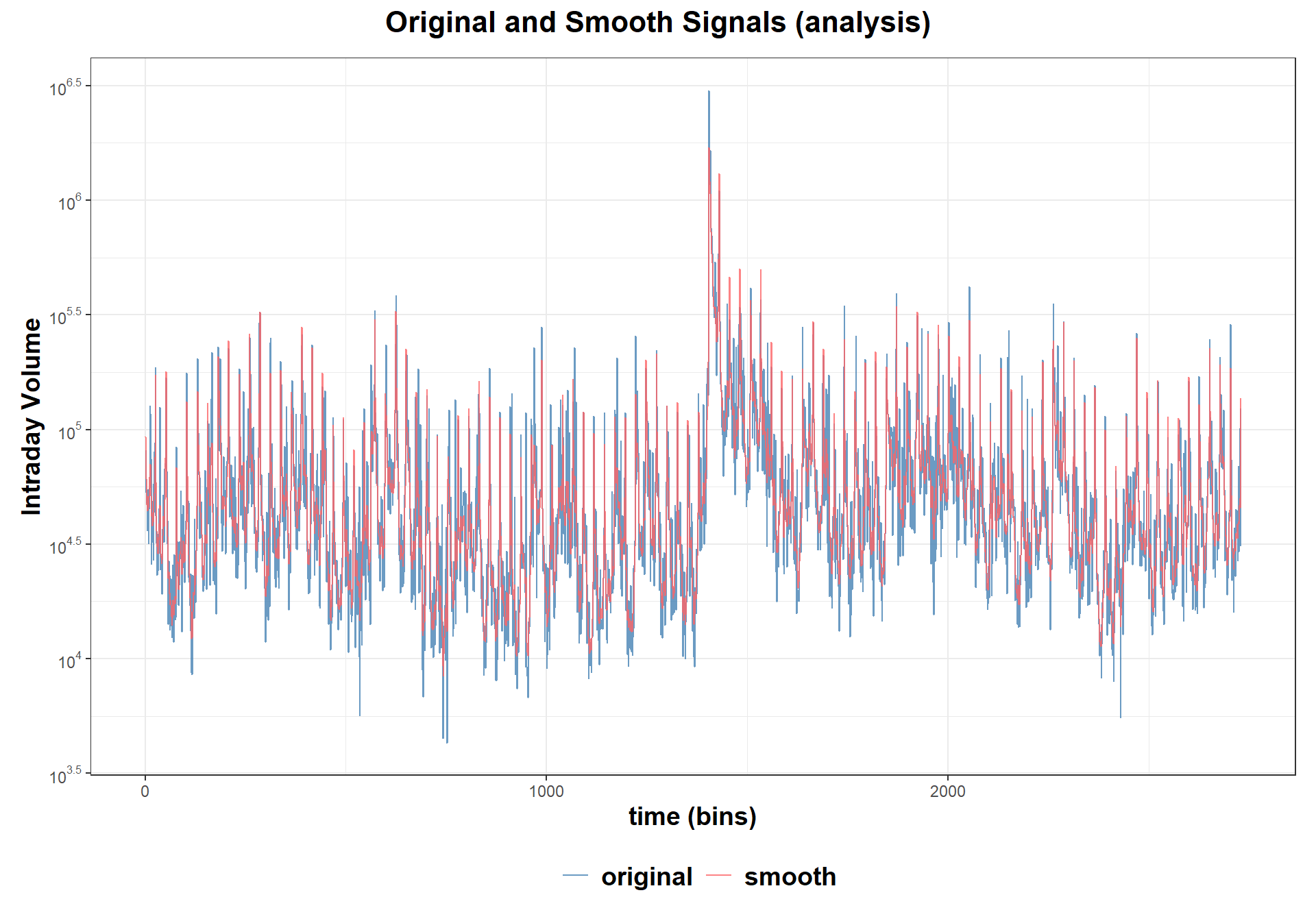With purpose = "forecast", it applies Kalman forecasting to estimate the one-bin-ahead hidden state based on the available observations, which is mathematically denoted by $$\mathbb{E}[\mathbf{x}_{\tau+1}|\{y_{j}\}_{j=1}^{\tau}]$$. Details can be found in the next subsection. This function also helps to evaluate the model performance with the following measures: • Mean absolute error (MAE): $$\frac{1}{M}\sum_{\tau=1}^M\lvert\hat{y}_\tau - y_\tau\rvert$$. • Mean absolute percent error (MAPE): $$\frac{1}{M}\sum_{\tau=1}^M\frac{\lvert\hat{y}_\tau - y_\tau\rvert}{y_\tau}$$. • Root mean square error (RMSE): $$\sqrt{\sum_{\tau=1}^M\frac{\left(\hat{y}_\tau - y_\tau\right)^2}{M}}$$. ## Forecasting forecast_volume(model, data, burn_in_days = 0) forecast_volume( ) function is a wrapper of decompose_volume(purpose = "forecast", ...). It forecasts the one-bin-ahead intraday volume on a new dataset. The one-bin-ahead forecast is mathematically denoted by $$\hat{y}_{\tau+1} = \mathbb{E}[y_{\tau+1}|\{y_{j}\}_{j=1}^{\tau}]$$. When encountering a new dataset with different statistical characteristics or from different stocks, the state space model may not initially start in an optimal state. To address this, the first burn_in_days days in the data can be utilized to warm up the Kalman filter, allowing it to reach the desired state. These initial days will be discarded after initialization. # use training data for burn-in forecast_result <- forecast_volume(model_fit, volume_fdx, burn_in_days = 105) #> Warning in intraday_xts_to_matrix(data): For input xts: #> Remove trading days with missing bins: 2019-07-03, 2019-11-29, 2019-12-24. str(forecast_result) #> List of 4 #>$ original_signal    : num [1:520] 149293 136426 134342 75474 61054 ...
#>  $forecast_signal : num [1:520] 81290 77773 94069 89915 72067 ... #>$ forecast_components:List of 4
#>   ..$daily : num [1:520] 37989 49345 57227 61320 59639 ... #> ..$ dynamic : num [1:520] 0.922 1.028 1.126 1.181 1.11 ...
#>   ..$seasonal: num [1:520] 2.32 1.53 1.46 1.24 1.09 ... #> ..$ residual: num [1:520] 1.837 1.754 1.428 0.839 0.847 ...
#>  $error :List of 3 #> ..$ mae : num 36242
#>   ..$mape: num 0.284 #> ..$ rmse: num 162071
#>  - attr(*, "type")= chr "forecast"

Function generate_plots( ) visualizes the one-bin-ahead forecast components and the forecasting performance.

plots <- generate_plots(forecast_result)
plots$log_components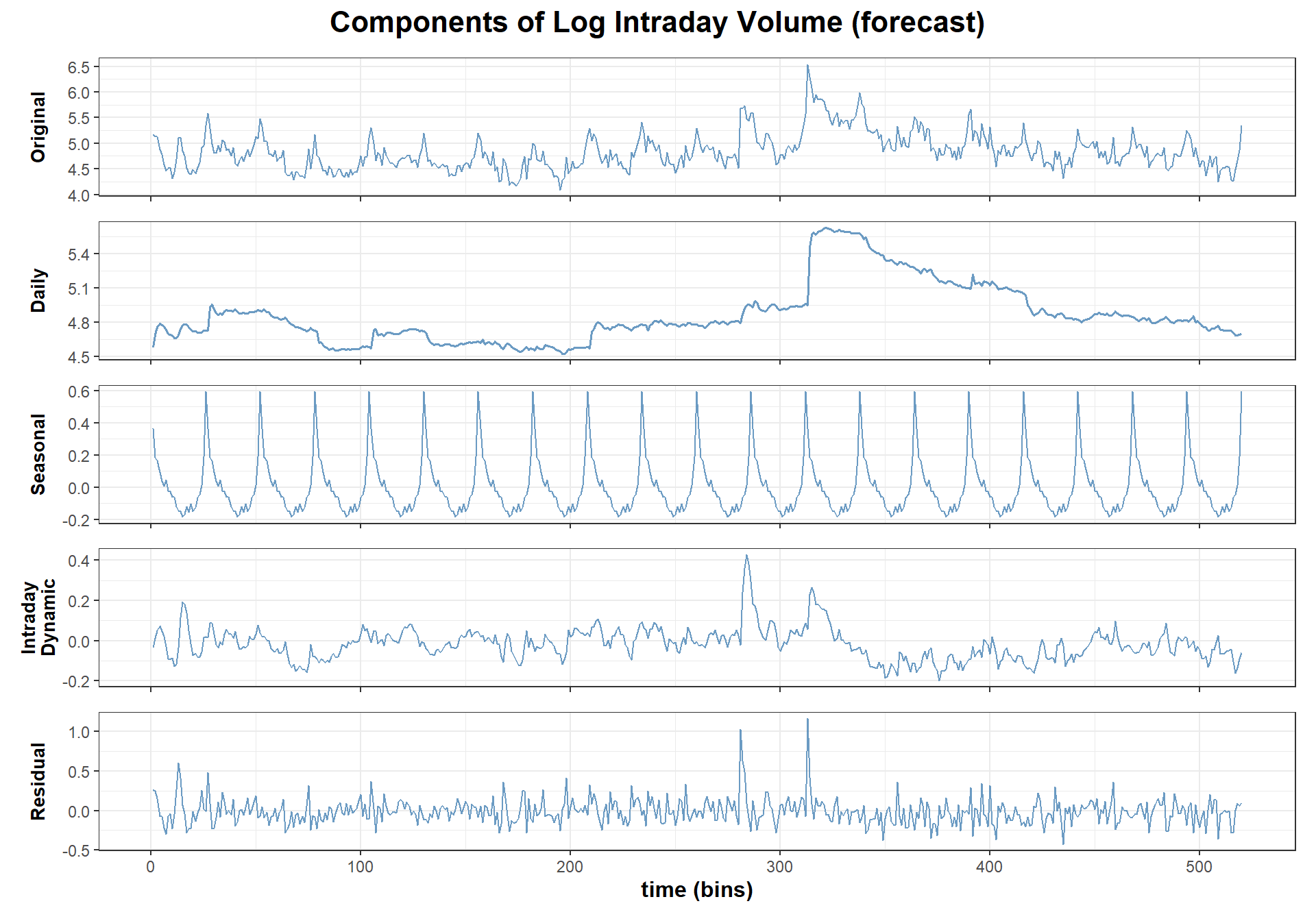plots$original_and_forecast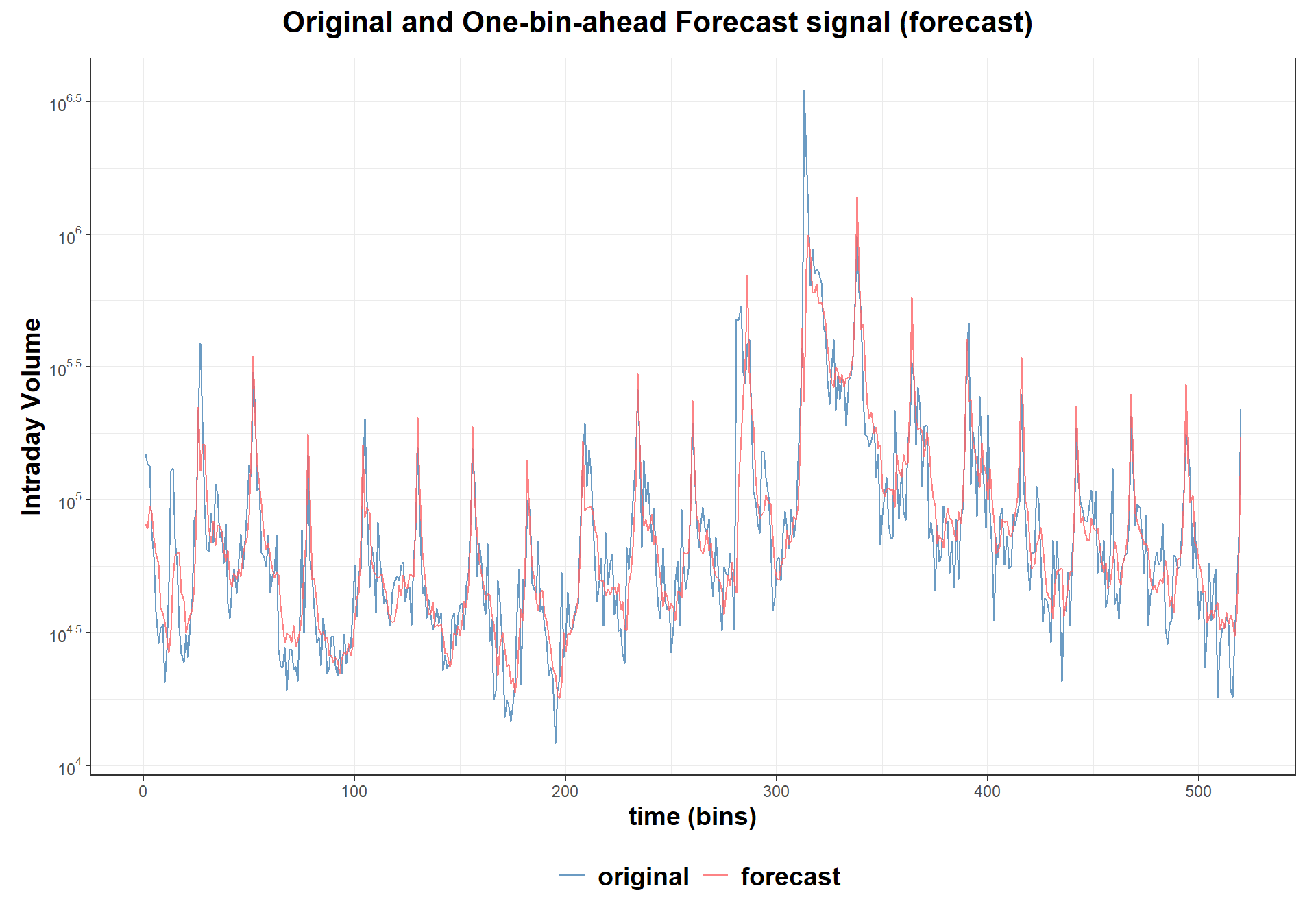# Next steps

This guide gives an overview of the package’s main features. Check the manual for details on each function, including parameters and examples.

The current version only supports univariate state-space models for intraday trading volume. Soon, we’ll add models for intraday volatility and their multivariate versions. We hope you find these resources helpful and that our package will continue to be a valuable tool for your work.

Brownlees, C. T., Cipollini, F., and Gallo, G. M. (2011). Intra-daily volume modeling and prediction for algorithmic trading. Journal of Financial Econometrics, 9(3), 489–518.
Chen, R., Feng, Y., and Palomar, D. (2016). Forecasting intraday trading volume: A Kalman filter approach. Available at SSRN 3101695.